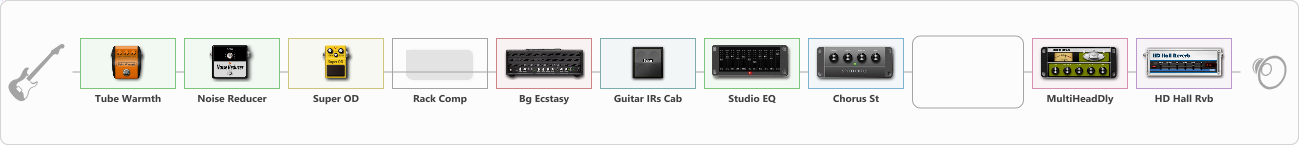# EVG

Discussion in 'ToneLib-GFX presets' started by Evgeniy ESP, Sep 3, 2022.

1. EVG

Preset name: bogner TIGHT

Effects chain:Effect: "Tube Warmth" (Dynamics / Filter), active - "yes"
{
"Input" = 30
"Warmth" = 74
"Level" = 50
}

Effect: "Noise Reducer" (Dynamics / Filter), active - "yes"
{
"Sens" = 57
"Mode" = Hard
}

Effect: "Super OD" (Овердрайв / Дисторшн), active - "yes"
{
"Drive" = 8
"Tone" = 80
"Level" = 100
}

Effect: "Rack Comp" (Dynamics / Filter), active - "no"
{
"Threshold (dB)" = -2
"Ratio" = 14
"Attack" = Fast
"Release (ms)" = 258
"Knee" = 46
"Level (dB)" = 0
}

Effect: "Bg Ecstasy" (Симуляторы усилителей), active - "yes"
{
"Gain" = 54
"Bass" = 26
"Middle" = 58
"Treble" = 50
"Presence" = 50
"Master" = 74
"Level (dB)" = 0
}

Effect: "Guitar IRs Cab" (Кабинеты), active - "yes"
{
"Model" = Marshall 1960A (4x12")
"Mic Position" = Center
"Mic Distance" = Near
"Low Cut (Hz)" = 75
"Hi Cut (kHz)" = 6.0
"Mix" = 100
"Level (dB)" = 0
}

Effect: "Studio EQ" (Dynamics / Filter), active - "yes"
{
"31 Hz" = 0
"62 Hz" = 0
"125 Hz" = 0
"250 Hz" = 0
"500 Hz" = 0
"1 kHz" = 0
"2 kHz" = 0
"4 kHz" = -6
"8 kHz" = 0
"16 kHz" = 0
"Level (dB)" = 0
}

Effect: "Chorus St" (Модуляция / Sfx), active - "yes"
{
"Speed" = 0.1
"Depth" = 0
"Center" = 4.4
"Mix" = 73
}

Effect: "Splitter" (Dynamics / Filter)
{
"A-Bypass" = Off
"A-Pan" = -25
"A-Level" = 55
"B-Bypass" = Off
"B-Pan" = 25
"B-Level" = 55
"Width" = 72

'A' branch:
{
}
'B' branch:
{
}
}

Effect: "MultiHeadDly" (Дилей), active - "yes"
{
"Time" = Синхронизация нот, 1/4
"Feedback" = 40
"Tone" = 34
"MODE" = Rhythm X-X-X-X
"Mix" = 40
}

Effect: "HD Hall Rvb" (Реверберация), active - "yes"
{
"Time" = 7.7
"PreLPF" = 4
"PreDelay" = 110
"HiDamp" = 27
"LoGain" = 2.0
"Mix" = 48
}

Note: You will need to download and install the ToneLib-GFX software to use the preset.

File size:
1 KB
Views:
1,548